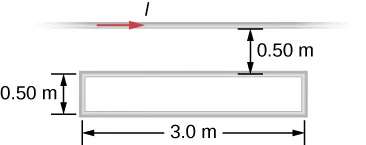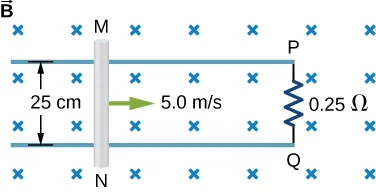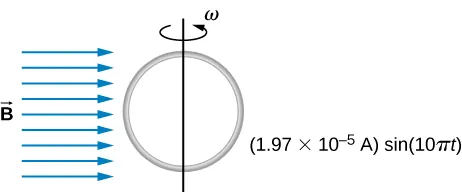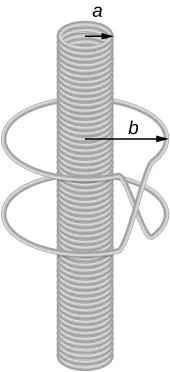University Physics Volume 2

63.

Shown in the following figure is a long, straight wire and a single-turn rectangular loop, both of which lie in the plane of the page. The wire is parallel to the long sides of the loop and is 0.50 m away from the closer side. At an instant when the emf induced in the loop is 2.0 V, what is the time rate of change of the current in the wire?64.

A metal bar of mass 500 g slides outward at a constant speed of 1.5 cm/s over two parallel rails separated by a distance of 30 cm which are part of a U-shaped conductor. There is a uniform magnetic field of magnitude 2 T pointing out of the page over the entire area. The railings and metal bar have an equivalent resistance of $150Ω.150Ω.$ (a) Determine the induced current, both magnitude and direction. (b) Find the direction of the induced current if the magnetic field is pointing into the page. (c) Find the direction of the induced current if the magnetic field is pointed into the page and the bar moves inwards.

65.

A current is induced in a circular loop of radius 1.5 cm between two poles of a horseshoe electromagnet when the current in the electromagnet is varied. The magnetic field in the area of the loop is perpendicular to the area and has a uniform magnitude. If the rate of change of magnetic field is 10 T/s, find the magnitude and direction of the induced current if resistance of the loop is $25Ω25Ω$.

66.

A metal bar of length 25 cm is placed perpendicular to a uniform magnetic field of strength 3 T. (a) Determine the induced emf between the ends of the rod when it is not moving. (b) Determine the emf when the rod is moving perpendicular to its length and magnetic field with a speed of 50 cm/s.

67.

A coil with 50 turns and area 10 $cm2cm2$ is oriented with its plane perpendicular to a 0.75-T magnetic field. If the coil is flipped over (rotated through $180°180°$) in 0.20 s, what is the average emf induced in it?

68.

A 2-turn planer loop of flexible wire is placed inside a long solenoid of n turns per meter that carries a constant current $I0I0$. The area A of the loop is changed by pulling on its sides while ensuring that the plane of the loop always remains perpendicular to the axis of the solenoid. If $n=500turnsn=500turns$ per meter, $I0=20A,I0=20A,$ and $A=20cm2,A=20cm2,$ what is the emf induced in the loop when $dA/dt=100?dA/dt=100?$

69.

The conducting rod shown in the accompanying figure moves along parallel metal rails that are 25-cm apart. The system is in a uniform magnetic field of strength 0.75 T, which is directed into the page. The resistances of the rod and the rails are negligible, but the section PQ has a resistance of $0.25Ω0.25Ω$. (a) What is the emf (including its sense) induced in the rod when it is moving to the right with a speed of 5.0 m/s? (b) What force is required to keep the rod moving at this speed? (c) What is the rate at which work is done by this force? (d) What is the power dissipated in the resistor?70.

A circular loop of wire of radius 10 cm is mounted on a vertical shaft and rotated at a frequency of 5 cycles per second in a region of uniform magnetic field of 2 Gauss perpendicular to the axis of rotation. (a) Find an expression for the time-dependent flux through the ring. (b) Determine the time-dependent current through the ring if it has a resistance of 10 $Ω.Ω.$71.

The magnetic field between the poles of a horseshoe electromagnet is uniform and has a cylindrical symmetry about an axis from the middle of the South Pole to the middle of the North Pole. The magnitude of the magnetic field changes as a rate of dB/dt due to the changing current through the electromagnet. Determine the electric field at a distance r from the center.

72.

A long solenoid of radius a with n turns per unit length is carrying a time-dependent current $I(t)=I0sin(ωt)I(t)=I0sin(ωt)$, where $I0I0$ and $ωω$ are constants. The solenoid is surrounded by a wire of resistance R that has two circular loops of radius b with $b>ab>a$ (see the following figure). Find the magnitude and direction of current induced in the outer loops at time $t=0t=0$.73.

A 120-V, series-wound dc motor draws 0.50 A from its power source when operating at full speed, and it draws 2.0 A when it starts. The resistance of the armature coils is $10Ω10Ω$. (a) What is the resistance of the field coils? (b) What is the back emf of the motor when it is running at full speed? (c) The motor operates at a different speed and draws 1.0 A from the source. What is the back emf in this case?

74.

The armature and field coils of a series-wound motor have a total resistance of $3.0Ω3.0Ω$. When connected to a 120-V source and running at normal speed, the motor draws 4.0 A. (a) How large is the back emf? (b) What current will the motor draw just after it is turned on? Can you suggest a way to avoid this large initial current?

Order a print copy

As an Amazon Associate we earn from qualifying purchases.